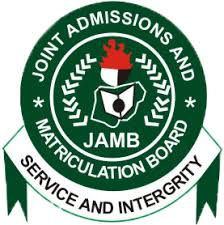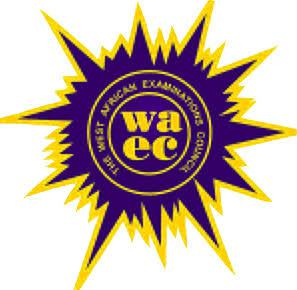HomeJambWaecNecoNabtebNews
Welccome To Examloaded.Com>>>>  Nigeria's No 1 Exam Expo Website, With 7 Years + of Experience Verified by Google #Na We Sure Pass!
Our Only Contact | Call | Text| WhatsApp08162563540

SUBSCRIBE HERE NOW!!# 2019/2020 Waec SSCE Physics Practical Expo Questions and Answers2019 WAEC SSCE PHYSICS PRACTICAL EXPO QUESTIONS AND ANSWERS

(1aviii)
In a tabular form
Under y(cm)
20.0, 30.0, 40.0, 50.0, 60.0

Under t(s)
36.61, 33.66, 31.32, 28.62, 25.93

Under T = t/20(s)
1.8305, 1.6830, 1.5660, 1.4310, 1.2965

Under T²(s²)
3.3507, 2.8325, 2.4524, 2.0478, 1.6809

(1ax)
Slope s = ΔT²/Δy
S = 4 – 2.5/0 – 39
S = 1.5/-39
S = -0.0385

Intercept, C = 4.0

(1axi)
SR = c
R = c/s = 4/-0.0385 = -103.9

(1axii)
(i) I ensured that the retort stand was well clamped.
(ii) I avoided error due to parallax when reading the metre rule.

(1bi)
Draw the diagram
(α) K.E is maximum
(β) acceleration is maximum

(1bii)
Period, T = 2π√m/k
Given : weight, W = 120N
Mass = w/g = 120/10 = 12
T = 45
π = 3.142
4 = 2(3.142)√12/k
0.6365 = √12/k
Square both sides
0.405 = 12
K = 12/0.405 = 29.6

PHYSICS PRACTICAL SPECIMEN:
Question 1
(1) Pendulum Bob
(2) Retort stand and clamp
(4) Stopwatch/clock
(5) Metre Rule
(6) Split cork.

Question 2
(1) Ray box with illuminated objects (cross wire)
(2) Convex lens(f = 15cm)
(3) Screen
(4) Metre rule.
(5) Lens Holder.

Question 3
(1) Battery
(2)Two dry cells(2×1.5v)
(3) Voltmeter.
(4) Resistor labeled R = 2Π
(5) Key
(6) Resistance box labelled R
(7) Seven connecting wires

## 2 Responses

1. I need it before 9 tomorrow

by ogechi on May 1, 2019 at 10:49 PM

2. pls i need math question

by kemi on May 7, 2019 at 3:51 PM

Theme by: EL Web Services